This tutorial shows different ways to add leading zeros in Excel: how to keep zeros as you type, show leading zeros in cells, remove or hide zeros.

If you use Excel not only to calculate numbers, but also maintain records such as zip codes, security numbers or employee ids, you may need to keep leading zeros in cells. However if you try typing a zip code like "00123" in a cell, Excel will immediately truncate it to "123".

The point is that Microsoft Excel treats postal codes, phone numbers and other similar entries as numbers, applies the General or Number format to them, and automatically removes preceding zeroes. Luckily, Excel also provides the means to keep leading zeros in cells, and further on in this tutorial you will find a handful of ways to do it.

## How to keep leading zeros in Excel as you type

For starters, let's see how you can put 0 in front of a number in Excel, for example type 01 in a cell. For this, simply change the cell format to Text:

• Select the cell(s) where you want to prefix numbers with 0.
• Go to the Home tab > Number group, and select Text in the Number Format box.

As soon as you type a zero(s) before number, Excel will display a small green triangle in the top-left corner of the cell indicating that something is wrong with the cell contents. To remove that error indicator, select the cell(s), click the warning sign, and then click Ignore Error.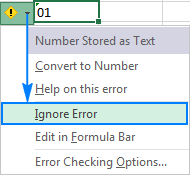The following screenshot shows the result: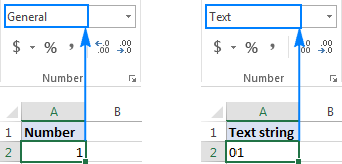Another way to keep leading zeros in Excel is to prefix a number with an apostrophe ('). For example, instead of typing 01, type '01. In this case, you don't need to change the cell's format.

Bottom line: This simple technique has a significant limitation - the resulting value is a text string, not number, and consequently it cannot be used in calculations and numeric formulas. If that is not what you want, then change only the visual representation of the value by applying a custom number format as demonstrated in the next example.

## How to show leading zeroes in Excel using a custom number format

To display leading zeroes, apply a custom number format by performing these steps:

1. Select a cell(s) where you want to show leading zeros, and press Ctrl+1 to open the Format Cells dialog.
2. Under Category, select Custom.
3. Type a format code in the Type box.

In most cases, you will need a format code consisting of 0 placeholders, like 00. The number of zeros in the format code corresponds to the total number of digits you want to show in a cell (you will find a few examples below).

4. Click OK to save the changes.

For example, to add leading zeros to create a 5-digit number, use the following format code: 00000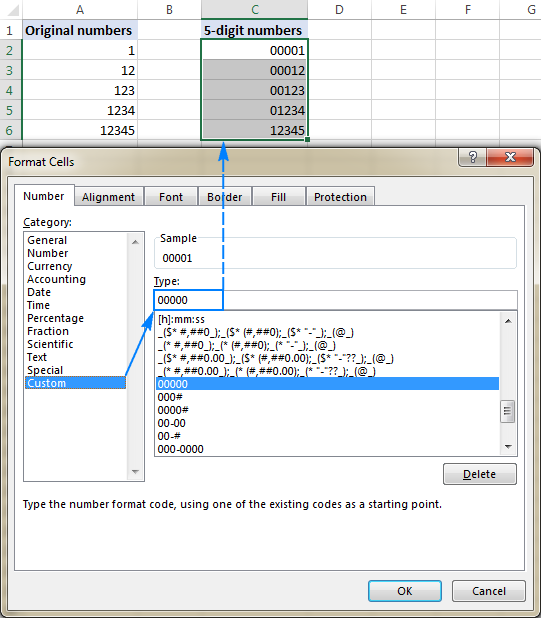By using Excel custom numbers formats, you can add leading zeros to create fixed-length numbers, like in the above example, and variable-length numbers. It all boils down to which placeholder you use in the format code:

• 0 - displays extra zeros
• # - does not display extra zeros

For example, if you apply the 000# format to some cell, any number you type in that cell will have up to 3 leading zeros.

Your custom number formats can also include spaces, hyphens, parentheses, etc. The detailed explanation can be found here: Custom Excel number format.

The following spreadsheet gives a few more examples of custom formats that can show leading zeros in Excel.

A B C
1 Custom format Typed number Displayed number
2 00000 123 00123
3 000# 123 0123
4 00-00 1 00-01
5 00-# 1 00-1
6 000-0000 123456 012-3456
7 ###-#### 123456 12-3456

And the following format codes can be used to display numbers in special formats such us zip codes, phone numbers, credit card numbers, and social security numbers.

A B C D
1   Custom format Typed number Displayed number
2 Zip code 00000 1234 01234
3 Social security 000-00-0000 12345678 012-34-5678
4 Credit card 0000-0000-0000-0000 12345556789123 0012-3455-5678-9123
5 Phone numbers 00-0-000-000-0000 12345556789 00-1-234-555-6789
Tip. Excel has a few predefined Special formats for postal codes, telephone numbers and social security numbers, as shown in the screenshot below: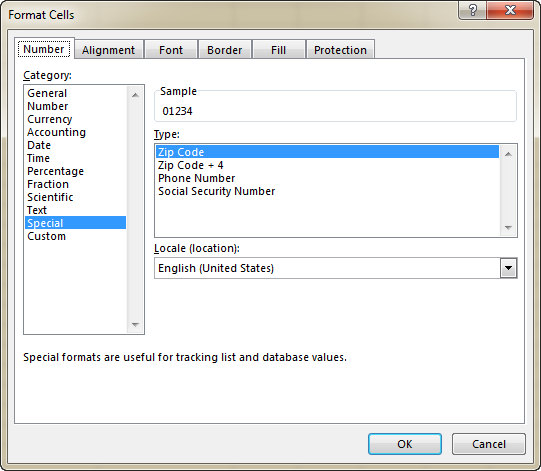Bottom line: This method is best to be used in situations when you work with a numeric dataset and the results should be numbers, not text. It changes only the display of a number, but not the number itself: leading zeros show up in cells, the actual value displays in the formula bar. When you reference such cells in formulas, the calculations are perfumed with the original values. Custom formats can only be applied to numeric data (numbers and dates) and the result is also a number or date.

## How to add leading zeros in Excel with the TEXT function

While a custom number format shows zero in front of a number without actually changing the underlying value, the Excel TEXT function pads numbers with zeros by "physically" inserting leading zeros in cells.

To add leading zeros with a TEXT(value, format_text) formula, you use the same format codes as in custom number formats. However, the result of the TEXT function is always a text string, even if it looks much like a number.

For example, to insert 0 before a value in cell A2, use this formula:

`=TEXT(A2, "0#")`

To create a zero-prefixed string of a fixed length, say a 5-character string, use this one:

`=TEXT(A2, "000000")`

Please pay attention that the TEXT function requires enclosing the format codes in quotation marks. And this is how the results will look like in Excel:

A B C
1 Original number Padded number Formula
2 1 01 =TEXT(B2, "0#")
3 12 12 =TEXT(B3, "0#")
4 1 00001 =TEXT(B4,"00000")
5 12 00012 =TEXT(B5,"00000")

Bottom line: The Excel TEXT function always returns a text string, not number, and therefore you won't be able to use the results in arithmetic calculations and other formulas, unless you need to compare the output with other text strings.

In the previous examples, you learned how to add zero before a number in Excel. But what if you need to put zero(s) in front of a text string like 0A102? In that case, neither TEXT nor custom format will work because they deal with numeric values only.

If the value to be padded with zero contains letters or other text characters, use one of the following formulas, which offer a universal solution applicable to both numbers and text strings.

The easiest way to put leading zeros before text strings in Excel is using the RIGHT function:

RIGHT("0000" & cell, string_length)

Where:

• "0000" is the maximum number of zeros you want to add. For example, to add 2 zeros, you type "00".
• Cell is a reference to the cell containing the original value.
• String_length is how many characters the resulting string should contain.

For example, to make a zero-prefixed 6-character string based on a value in cell A2, use this formula:

`=RIGHT("000000"&A2, 6)`

What the formula does is add 6 zeros to the value in A2 ("000000"&A2), and then extract the right 6 characters. As the result, it inserts just the right number of zeros to reach the specified total string limit: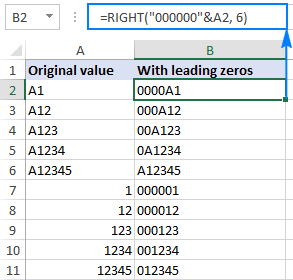In the above example, the maximum number of zeros equals the total string length (6 characters), and therefore all of the resulting strings are 6-character long (fixed length). If applied to a blank cell, the formula would return a string consisting of 6 zeros.

Depending on your business logic, you can supply different numbers of zeros and total characters, for example:

`=RIGHT("00"&A2, 6)`

As the result, you will get variable-length strings that contain up to 2 leading zeros: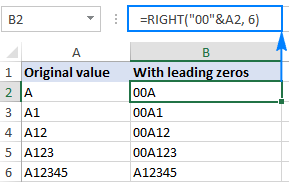### Formula 2. Pad leading zeros using the REPT and LEN functions

Another way to insert leading zeros before a text string in Excel is using this combination of REPT and LEN functions:

REPT(0, number of zeros-LEN(cell))&cell

For example, to add leading zeroes to the value in A2 to create a 6-character string, this formula goes as follows:

`=REPT(0, 6-LEN(A2))&A2`

#### How this formula works:

Knowing that the REPT function repeats a given character a specified number of times, and LEN returns the total length of the string, the formula's logic is easy to understand:

• LEN(A2) gets the total number of characters in cell A2.
• REPT(0, 6-LEN(A2)) adds the required number of zeros. To calculate how many zeros should be added, you subtract the length of the string in A2 from the maximum number of zeros.
• Finally, you concatenate zeros with the A2 value, and get the following result: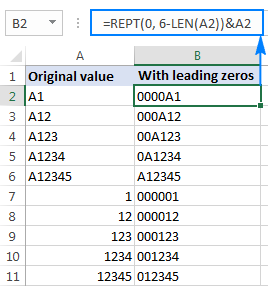Bottom line: This formula can add leading zeros both to numbers and text strings, but the result is always text, not number.

## How to add a fixed number of preceding zeros

To prefix all values in a column (numbers or text strings) with a certain number of zeros, use either the CONCATENATE function or the ampersand operator.

For example, to put 0 before a number in cell A2, use one of these formulas:

`=CONCATENATE(0,A2)`

or

`=0&A2`

As shown in the screenshot below, the formula adds just one leading zero to all cells in a column regardless of how many characters the original value contains: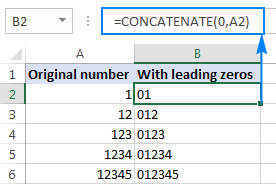In the same manner, you can insert 2 leading zeros (00), 3 zeros (000) or as many zeros as you want before numbers and text strings.

Bottom line: The result of this formula is also a text string even when you are concatenating zeros with numbers.

## How to remove leading zeros in Excel

The method you use to remove leading zeros in Excel depends on how those zeros were added:

• If preceding zeroes were added with a custom number format (zeros are visible in a cell, but not in the formula bar), apply another custom format or revert back General as shown here.
• If zeros were typed or otherwise entered in cells formatted as Text (a small green triangle is displayed in the top-left corner of the cell), convert text to number.
• If leading zeroes were added by using a formula (the formula appears in the formula bar when the cell is selected), use the VALUE function to remove them.

The following image shows all three cases to help you choose the right technique: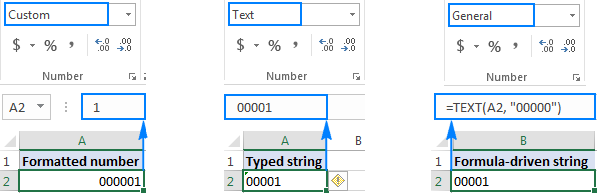### Remove leading zeros by changing the cell format

If leading zeroes are shown in cells with a custom format, then change the cell format back to default General, or apply another number format that does not display preceding zeros.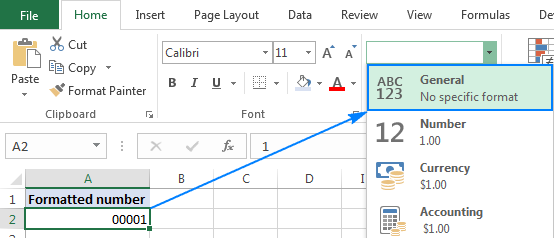### Remove leading zeros by converting text to number

When prefixed zeros appear in a Text-formatted cell, the easiest way to remove them is select the cell(s), click the exclamation point, and then click Convert to Number: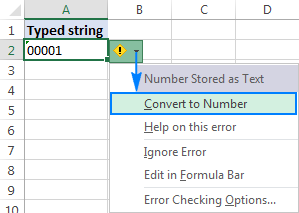### Remove leading zeros by using a formula

In case a preceding zero(s) is added with a formula, use another formula to remove it. The zero-removing formula is as simple as:

`=VALUE(A2)`

Where A2 is the cell from which you want to remove preceding zeros.

This method can also be used to get rid of zeros typed directly in cells (like in the previous example) or imported to Excel from some external source. Overall, if you are dealing with a zero-prefixed string that represents a number, you can use the VALUE function to convert text to number and remove leading zeros along the way.

The following screenshot shows two formulas:

• The Text formula in B2 adds zeros to the value in A2, and
• The Value formula in C2 removes the leading zeros from the value in B2.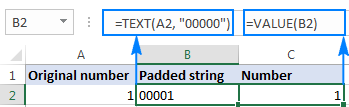## How to hide zeros in Excel

If you don't want to display zero values in your Excel sheet, you have the following two options:

1. To hide zeros across the entire sheet, uncheck the Show a zero in cells that have zero value option. For this, click File > Options > Advanced, and scroll down to the Display options for this worksheet section: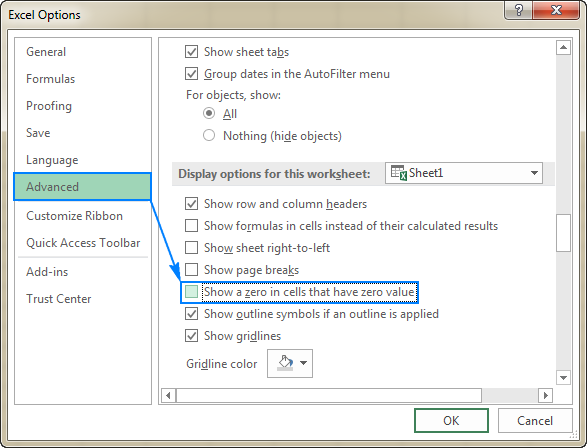2. To hide zero values in certain cells, apply the following custom number format to those cells: #;#;;@

For this, select the cells where you want to hide zeros, click Ctrl+1 to open the Format Cells dialog, select Custom under Category, and type the above format code in the Type box.

The screenshot below shows that cell B2 does contain a zero value, but it is not displayed in the cell: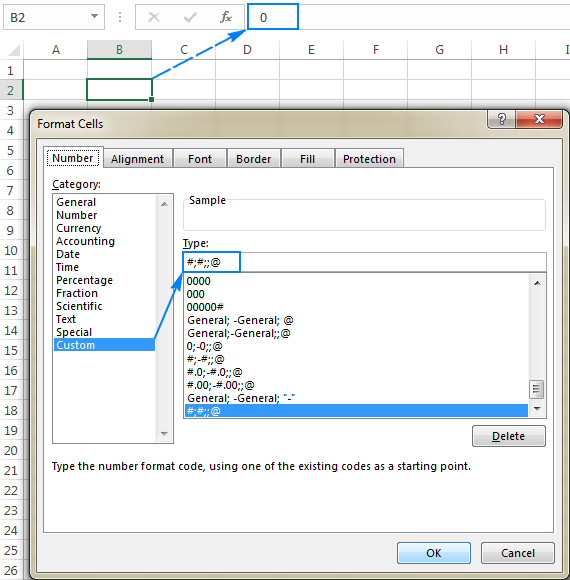This is how you can add, remove and hide zeros in Excel. To better understand the techniques described in this tutorial, you are welcome to download the sample Excel Leading Zeros Workbook. I thank you for reading and hope to see you on our blog next week.

### You may also be interested in:

1.Imran Masud says:

Lots of thanks. Keep on.

2.Rohan Singh says:

WOW

•Agant Patil says:

Fantastic!

3.MSVCP140 says:

:-) OK !

4.prakash Bk says:

kindly let us know how add in exl before 0
like 01233

5.Somnath says:

Just put '

6.prakash Bk says:

wow

7.Farshid says:

Flawless,

8.Mohamed says:

i want this number 095009419000000 to 95009419
and this number 095094100090000000 to this 9509410009

9.Dinis says:

Thank you very much!

10.tim streets says:

In my job, I have to type in nhs numbers on a spreadsheet. The old numbers were 6 digits, the new 8 digits. Logically, the first numbers (6 or 8 digits) began with a zero, until they passed the 099999 or 09999999. How can I display them in their true format, without formatting them as text? I have tried custom formats such as"00000000", but it still puts 2 zeros in front of a 6 digit number

•Natalia Sharashova (Ablebits.com Team) says:

Hi, Tim,

you need to customize your number formats. Here we have a good tutorial on the process. The format code for you to use there:
[>999999]00000000;[<=999999]000000

11.Abdul says:

Thanks for the lesson.

Please, is there a way one can retain the zeros in front of mobile numbers after the cell has been formatted and the mobile numbers copied and pasted instead of entering them one after the other?

I'm trying to convert them to VCard Files but the numbers copied and pasted do not have the zeros in front of them after conversion unlike the ones that were entered individually.

I will greatly appreciate your prompt response.

Thank you.

12.Ajay says:

i want to calculate how many zero in one cell can anyone help me in that for example i write 5000 in one cell in 5000 there is 3 zero in that figure.

13.CGent says:

I want 5.25 to be 0-052 is this possible?

14.Bassam says:

Hi I have different numbers in row just now wants to add 0 before which starts with 4 how can we do that

15.Jessie says:

Best solution.

16.AJEET says:

Hi I have subtracts some number and generate a series and wants a suffix in this series.
i.e.
16 RR
13 RR
10 RR

17.Ahmed says:

Hii
i want to remove the extra zeros after the percentage to be 95% instead of 9500%

•Doug says:

Ahmed:
Can you initially enter the number as .095 then format it as a percentage? Right now, it's entered as 95 and then formatted as a percentage.

18.Rajesh says:

When entering 17 digits of RSBY, it shows last two digit as 00. Please guide for converting it to its original number. Eg: 32245614656464567, it shows 32245614656464500. Please guide me to correct this option.

19.Tasneem says:

How should i enter the numbers in excel into ID format. For instance 17775608 should appear as 0000-0000-1777-5608

•Svetlana Cheusheva (Ablebits.com Team) says:

Hi Tasneem,

Apply this custom number format: 0000-0000-0000-0000

20.DataScientist says:

Instructions are clear and detailed and with plenty of illustrations. Great job, Svetlana!

21.Emmanuel says:

Hi,
I want to add zero and space e,g (078 444 7777). But every time i add number it show like this (0784447777).

22.Christoffer Thrane says:

I love the internet for this reason! thanks for helping out

23.Steve says:

I really need some help, i have time stamps (6:25) which is minutes and seconds but i need it to show the hour without having to manually ad the 0: in the front (0:06:25) How can i make 6:25 to show 0:06:25.

24.maheshworsapkota says:

how to bank loan no create in excel sheet like 2121254000000000000000001111111111111

25.JORGE FERNANDES says:

I have a Number R00835 instead I need R0835.How to supress one zero.
Jorge Fernandes

•JC says:

=SUBSTITUTE(TRIM(SUBSTITUTE(YOURFIELD,"0"," "))," ","0")

26.Sri says:

Hello all,
How to convert a number with only two decimal places?
For example:
If I type 10, it should come as 10.000
If I type 100, it should come as 100.00
How to do it? Thanks in advance.

27.Jo says:

Hi
I need a list of numbers to all be 10 digits with leading zeros making up the 10 digits.
Original numbers can have different numbers of digits eg they could be 15153, 178013.
These would need to be 0000015153, 0000178013
I can see solutions here to always add one, or two digits, but not how to add a dynamic number of leading digits depending on the how many digits the number already has.
Do you have any solution for this?
Thanks

28.Danny says:

hi
i have downloaded a excell file of telephone numbers to edit and then upload to a telephone system.
it has removed the leading 0 from all telephone numbers. i need to put the 0 back but keep the format as text. i have tried TEXT(value, format_text) but because its a 11 digit telephone number its saying there is a maximum of 20 digits.

Can anyone help

29.Rex says:

how to remove in phone number?
here's the example phon number 0417 628 651 .i need to remove zero and this is the 417628651 ...

30.Emily says:

This saved me an outrageous amount of time. Thank you so much!

31.Larry says:

it want to combine year month and date columns to the following:
2019-06-30

I can get the month col as 06, but When I put it into the date format with the ampersand,
32.Farid says: